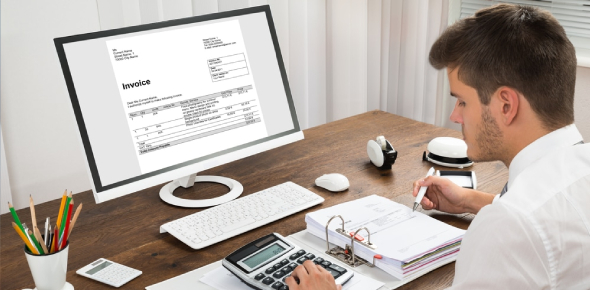# Basic Accounting MCQ Exam: Quiz!

10 Questions | Total Attempts: 300SettingsWhat do you know about basic accounting? Could you pass an exam? If you are not sure, this quiz can help. Basic accounting refers to the process of recording a company’s financial exchanges. It involves analyzing, summarizing, and reporting these exchanges to tax collection agencies. The primary assets of a business include cash, accounts receivable, inventory, and fixed assets. If you want to put your knowledge to the test, this quiz is above all the rest.

• 1.
Net profit is calculated in?
• A.

Balance Sheet

• B.

Profit and Loss Account

• C.

• D.

Trial Balance

• 2.
Fixed assets can be described as?
• A.

Furniture

• B.

Cash at Bank

• C.

Debtor

• D.

Bank Loans

• 3.
Drawings are when the owner
• A.

• B.

Gives money to charity

• C.

Takes out business money for personal use

• D.

Reduces the employee's pay

• 4.
Assets increases on the debit side.
• A.

True

• B.

False

• 5.
Fixed asset is bought by the business for?
• A.

Resale

• B.

Loans

• C.

• D.

Owner's use

• 6.
The accounting equation is ?
• A.

Assets - Liability = Owner's equity

• B.

Liability = Owner's equity = Assets

• C.

Assets + Liability = Owner's equity

• D.

Currents Assets = Long-term liability - Owner's equity

• 7.
Trial Balance is done after T-accounts.
• A.

True

• B.

False

• 8.
The company makes a loss when the amount spent on the purchases is lower than the sale.
• A.

True

• B.

False

• 9.
What is another word for revenue?
• A.

Income

• B.

Expenses

• C.

Liability

• D.

Assets

• 10.
Gross profit is calculated in the?
• A.

T- Accounts

• B.

Profit and Loss Account

• C.Back to top